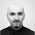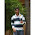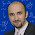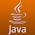Thursday, September 22, 2011

Replacing the Java random generator

The Java random number generator was implemented in 1995. It is based on a linear congruential generator. Since then, there was considerable advancement in algorithms for pseudo random number generators. For most applications, Java's random number generator might be sufficient, but at a closer look, the algorithm has a fairly short period of 248 and fails some tests on the randomness of its output.
The good news is that there is an algorithm which is faster and better: the Xorshift algorithm. Xorshift is using a few (in the following implementation exactly three) of shift and exclusive-or operations. The best way to integrate it to Java is to make a subclass of java.util.random and to overwrite the `seed` variable and the `next()` method:

``````import java.util.Random;

/**
* A subclass of java.util.random that implements the
* Xorshift random number generator
*/

public class XSRandom extends Random {
private long seed;

public XSRandom(long seed) {
this.seed = seed;
}

protected int next(int nbits) {
long x = seed;
x ^= (x << 21);
x ^= (x >>> 35);
x ^= (x << 4);
seed = x;
x &= ((1L << nbits) - 1);
return (int) x;
}
}
``````

Since all methods of the Random generator (`nextBoolean()`, `nextInt()`, `nextLong()`, `nextFloat()`, `nextDouble()`), `nextBytes()`, `nextGaussian()`) depend on the `next()` method, this efficiently changes all number generation to the Xorshift algorithm.
A complete Java class including also a `clone()` method can be downloaded here (Code is under LGPL Version 3). Note that this implementation, unlike the `java.util.Random` is not exactly thread-safe - concurrent threads might access and change the seed variable inconsistently. However, note that concurrent access on the same random object would anyway end up in a nondeterminstic sequence of numbers for each thread.
This implementation is about 30% faster than the generator from java.util.random. It's output passes the Dieharder test suite with no fail and only two announced weaknesses. To use the class in legacy code, you may also instantiate an `XSRandom` object and assign it to a java.util.Random variable:
``  java.util.Random rand = new XSRandom();``
All method calls to rand are then using the newer, faster implementation.

1.I have no words for this great post such a awe-some information i got gathered. Thanks to Author.
Vee Eee Technologies| Vee Eee Technologies|

2.Found a problem with your code

currentSeed &= ((1L << bits) - 1);

Eventually you will max out the long causing nothing but the max value of long to be sent

instead remove that line and change the return statement to

return (int) (currentSeed & ((1L << bits) - 1));

1.Not a problem in the current version:
x &= ((1L << nbits) - 1);
will return at most x (because of the '&')

3.Hi I am Pari,
Will this Algorithm produces sequence no too ?
If no then anyother way to produce sequence no in java?

Thanks

1.Do you mean generating random numbers out of a set of sequences numbers? Then you can use the proposed algorithm above together with the algorithm proposed here: http://stackoverflow.com/questions/20372416/generate-random-numbers-increasing-by-multiples-of-n-in-java

4.xorshift(0) == 0, so "xs = new XSRandom(0)" will only output zero.
You need either to test against zero or to use a bigger xorshift variant, such as http://xorshift.di.unimi.it/

5.Nice Example Visit More Java Example